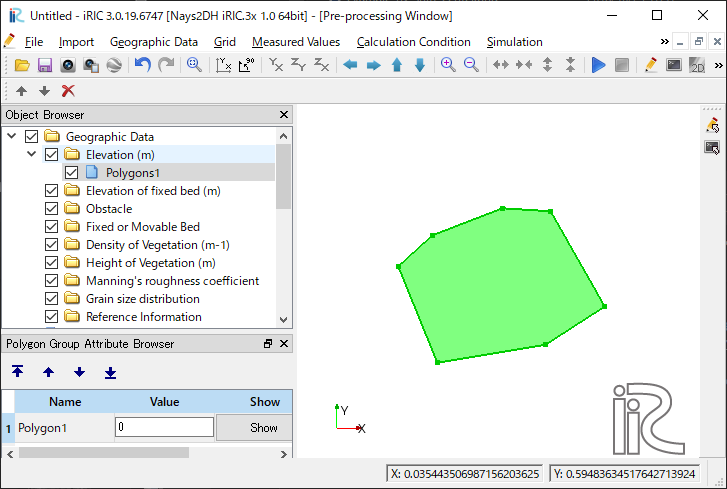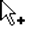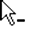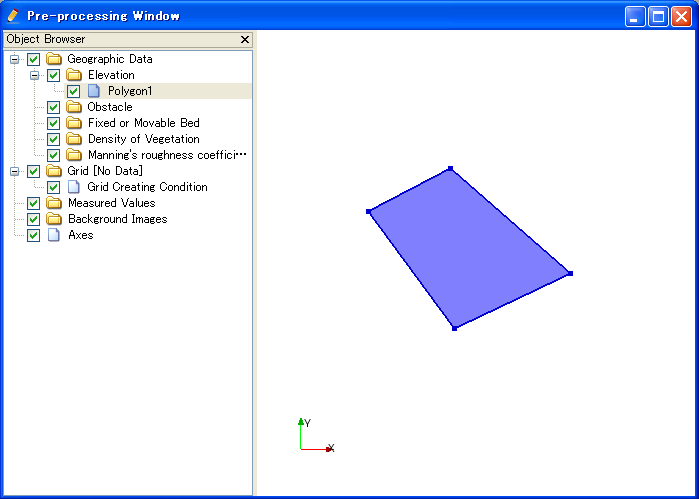Editing the [Polygon]¶

Description: Sets the values of geographic data within the area of the Polygon. Figure 193 shows an example of the [Polygon].

The procedure to add a new Polygon is as follows:

1. Select the type of [Geographic Data] in [Object Browser] to which you want to add a Polygon (Figure 194). Note that the list of types of [Geographic Data] varies according to the solver used.
2. The operation below adds a new Polygon to [Object Browser], and that Polygon is selected.

Menu bar: [Geographic Data] (E) –> [Polygon] (P) –> [Add New Polygon]

1. On the canvas, add vertexes to the Polygon by left clicking (Figure 195).
2. Double click or press the Enter key to complete defining the Polygon. As soon as you have double clicked or pressed the Enter key, the [Edit Elevation value] dialog will open(Figure 196). Set the geographic data of the Polygon and click on [OK]. The dialog varies depending on the type of [Geographic Data] to which a Polygon is added.Figure 195 [Pre-processing Window] when the [Polygon] is being defined

[Edit Value] (V)¶

Description: Edits data value within the Polygon.

When you select [Edit Value], the [Edit Elevation value] dialog (Figure 197) will open. Set a new value and click on [OK].

[Copy]¶

Description: Copy the Polygon to another [Geographic Data] group.

When you select [Copy], the [Select Geographic Data] dialog (Figure 198) will open. Select the [Geographic Data] group to copy this polygon, and click on [OK].

Then, the dialog to specify the value for the polygon is shown, like in Figure 199. Specify the value and click on [OK], to finish copying the polygon.

Description: Adds a vertex to the [Polygon].

When you select [Add Vertex] and move the cursor to the edge of [Polygon], the cursor changes to that shown in Figure 200. Left click on the line and drag it to add a new vertex. The vertex is placed where you release the left button.Figure 200 Mouse cursor when possible to add a vertex

[Remove Vertex] (R)¶

Description: Deletes a vertex from the [Polygon].

When you select [Remove Vertex] and move the cursor onto the vertex you want to remove, the cursor changes to that shown in Figure 201. Left clicking will remove the vertex.Figure 201 Mouse cursor when possible to removing the vertex

[Edit Coordinates] (C)¶

Description: Edits the coordinates of the vertices of the [Polygon].

When you select [Edit Coordinates], the [Polygon Coordinates] dialog (Figure 202) will open. Edit the coordinates and click on [OK].

[Add Hole Region] (H)¶

Description: Adds the Hole Region to the [Polygon].

1. When you select [Add Hole Region], the [Information] dialog (Figure 203) will open. Click on [OK].
2. On the canvas, add vertexes to the Hole Region by left clicking (Figure 204).
3. Double click or press the Enter key to complete defining the Hole Region.

[Delete Hole Region] (D)¶

Description: Deletes the Hole Region from the [Polygon].

1. When you select [Delete Hole Region], the [Warning] dialog (Figure 205) will open. Click on [Yes].
2. On the canvas, the Hole Region will be deleted. (Figure 206).Figure 206 Example of the [Delete Hole Region] Result

[Color Setting] (S)¶

Description: Edits the color of the [Polygon].

When you select [Color Setting], the [Polygon Color] dialog (Figure 207) will open. Set it and click on [OK].